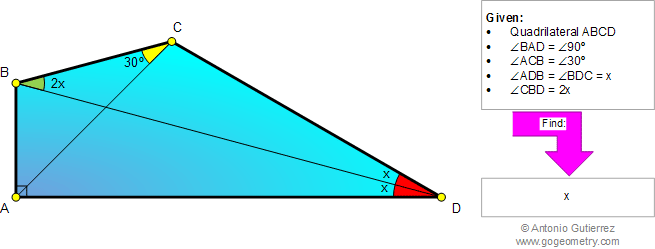Online Geometry Problem 872: Quadrilateral, Triangle, Angle, 30, 90 degrees. Level: High School, College, Mathematics Education
 The figure below shows a quadrilateral ABCD with angle BAD = 90 degrees, angle ACB = 30 degrees, angle ADB = angle BDC = x, and angle CBD = 2x. If angle BCD is obtuse, find x.Home | Search | Geometry | Problems | All Problems | Open Problems | Visual Index | 10 Problems | 871-880 | Triangles | Quadrilateral | Angles | 30 Degrees | Email | Solution / comment | By Antonio Gutierrez